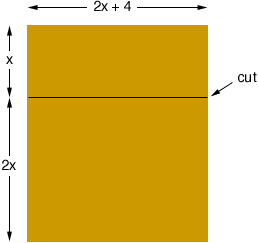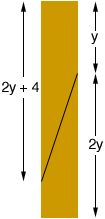Quandaries and Queries George Grade 11 Student A person has a sheet of board which he saws into two (2) pieces. The length of the first piece is two thirds the length of the original board, while the length of the second piece is four feet longer than the first piece. How long was the original board? (the length is defined as the longer of the two sides of the board) Hi George, I see two ways of interpreting this problem. One: The cut is perpendicular to one side of the sheet Here a diagram would beIn this diagram the original board is 3x feet long. If the original board was square then 3x = 2x + 4 and x = 4 feet, and the original board was 12 feet long. But you do not need the board to be square. x might be 8 feet and then the original baord is 24 feet by 20 feet. There are in fact infinitely many solutions. Two: The cut is not perpendicular to one side of the sheet Here a diagram might beHere y might be 8 feet making the original board 24 feet long. But y might also be 5 feet and then the original baord would be 15 feet long. Again there are infinitely many solutions. I hope this helps, Penny Go to Math Central